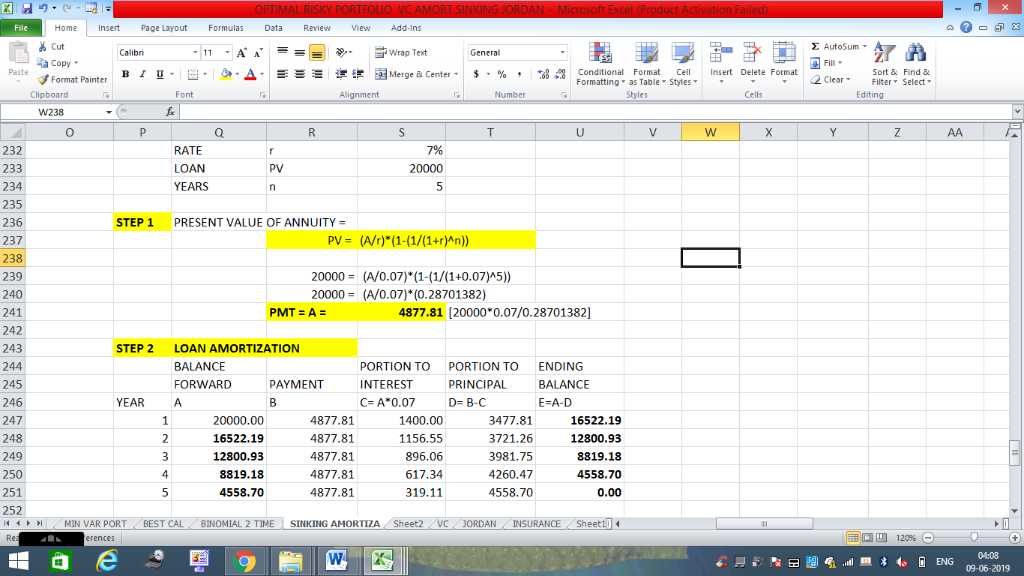In: Finance

# An investor plans to borrow $20,000. The loan will be repaid in 5 annual payments. The... An investor plans to borrow$20,000. The loan will be repaid in 5 annual payments. The interest rate charged is 7%. Find the loan payment and amortize the loan

Use formulas and show step by step with calculations.

## Solutions

##### Expert Solution## Related Solutions

A loan of 10000$is to be repaid with annual payments, at the end of each year, for the next 20 years. For the rst 5 years the payments are k per year ; the second 5 years, 2k per year ; the third 5 years, 3k per year ; and the fourth 5 years, 4k per year. (a) Draw two timelines describing this series of payments. (b) For each of the timelines in (a), find an expression for k... ##### A loan of$5000 is repaid with annual payments at the end of each year of...
A loan of $5000 is repaid with annual payments at the end of each year of$1200,$800,$1300 and X. Assume the loan has 10% effective interest per year. a) Determine X b) Determine the amount of interest paid with the third payment.
##### Donald takes out a loan to be repaid with annual payments of $500 at the end... Donald takes out a loan to be repaid with annual payments of$500 at the end of each year for 2n years. The annual effective interest rate is 4.94%. The sum of the interest paid in year 1 plus the interest paid in year n + 1 is equal to $720. Calculate the amount of interest paid in year 10. ##### A loan of$10,000 is to be repaid with 10 semi-annual payments. The first payment is...
A loan of $10,000 is to be repaid with 10 semi-annual payments. The first payment is X in 6 months time. i(2) = 4%. Find X if a) Payments increase by$100 every 6 months. b) Payments increase by 10% every 6 months.
A loan will be repaid in 5 years with monthly payments at a nominal interest rate of 9% monthly convertible. The first payment is $1000 and is to be paid one month from the date of the loan. Each succeeding monthly payment will be 2% lower than the prior payment. Calculate the outstanding loan balance immediately after the 40th payment is made. ##### FINANCIAL MATH QUESTION 1 The loan of$ 20,000, received at 9% annual interest, is repaid...
FINANCIAL MATH QUESTION 1 The loan of $20,000, received at 9% annual interest, is repaid as follows: (a) after 2 months$ 5,000, (b) after 5 months, the amount X, (c) after 9 months \$ 8,000. How much to pay after 5 months? Interest is calculated on the balance of the debt. Time calculation method "30/360".
##### James has a loan of 10,000, which is to be repaid with 10 level annual payments...
James has a loan of 10,000, which is to be repaid with 10 level annual payments at an annual effective interest rate of 14.5%. Calculate the Macaulay duration of the loan using the 14.5% interest rate.